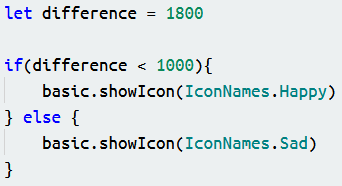Quiz - Introduction to JavaScript
What is JavaScript? *
10 points
Do we use code blocks or typed code in JavaScript? *
10 points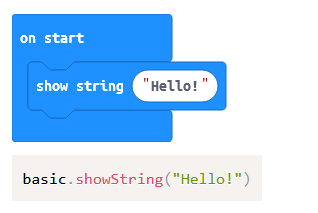What is a sequence? *
10 points
If we were programming a Microbit using this JavaScript code, what would the code make the Microbit do? *
10 points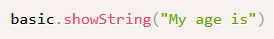If we were programming a Microbit using this JavaScript code, what would the code make the Microbit do first? *
10 points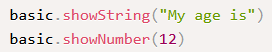There is a problem with this code and it will not work. Which of the following is the correct code? *
10 points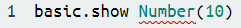What is this JavaScript code doing? *
10 points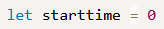What does putting // in your JavaScript code do? *
10 points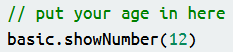What icon will this code show? *
10 points